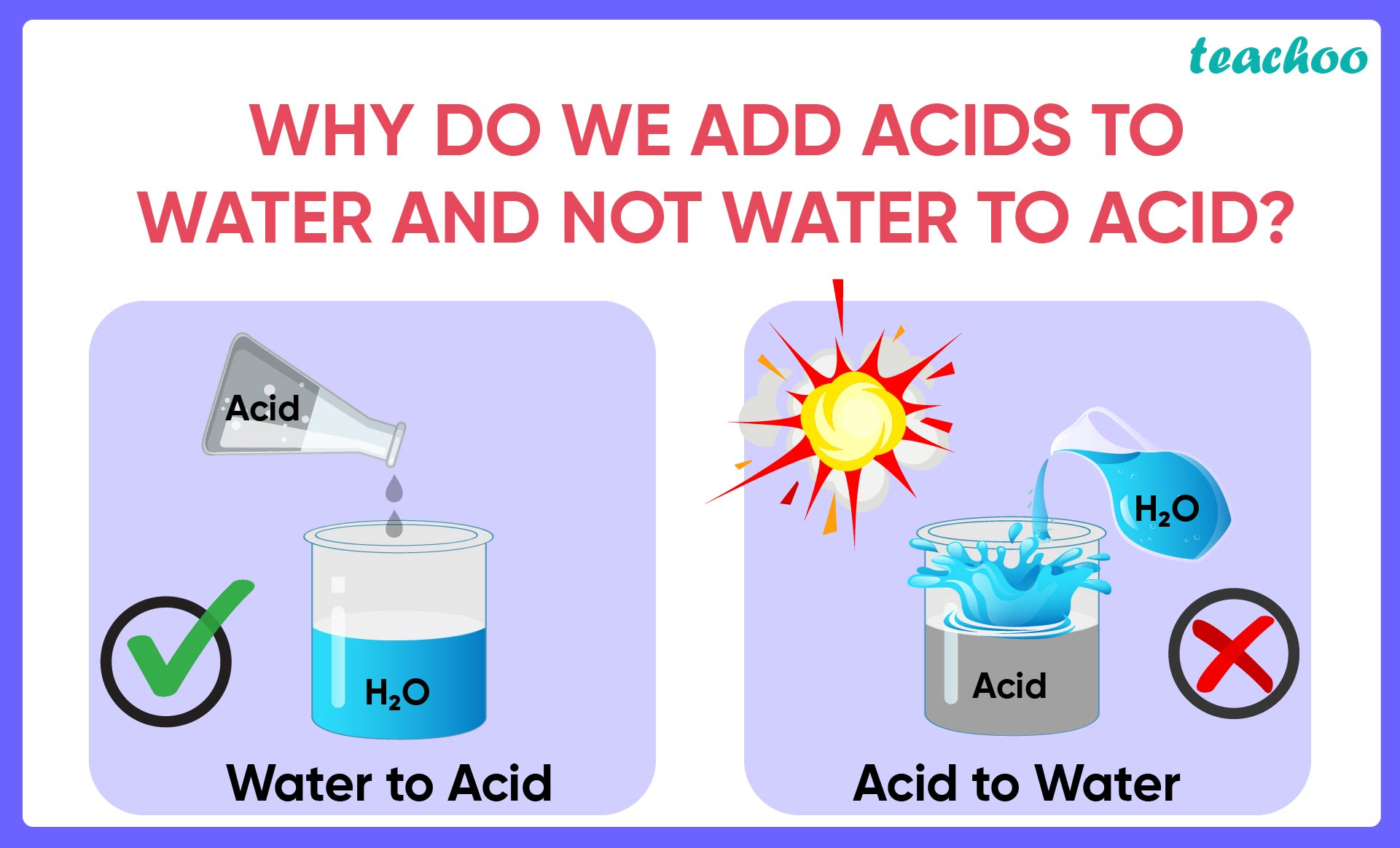Assertion Reasoning Questions (MCQ)

Class 10
Chapter 2 Class 10 - Acids, Bases and Salts (Term 1)

## Reason (R): Specific heat of water is quite large.

### (d) Assertion (A) is false but reason (R) is true.Now,

• The mixing of water to an acid is  highly exothermic in nature.
• If water is added to an  acid it produces a very large amount of heat. This  can break the container and sometimes even causes burning as acids can heat up very quickly.
• So it is advised to add concentrated acid  to water in a very slow manner.

Also,

• Specific heat capacity is the amount of heat needed to raise one gram of a material by one degree celsius.
• Specific heat of water is high as compared to other materials

Since the specific heat of water is high , it takes a lot of heat to increase its temperature. Thus, we add acid to water and not water to acid

Thus,

• Assertion is true
• Reasoning is true
• And Reasoning is the correct explanation of Assertion

So, the correct answer is (a)